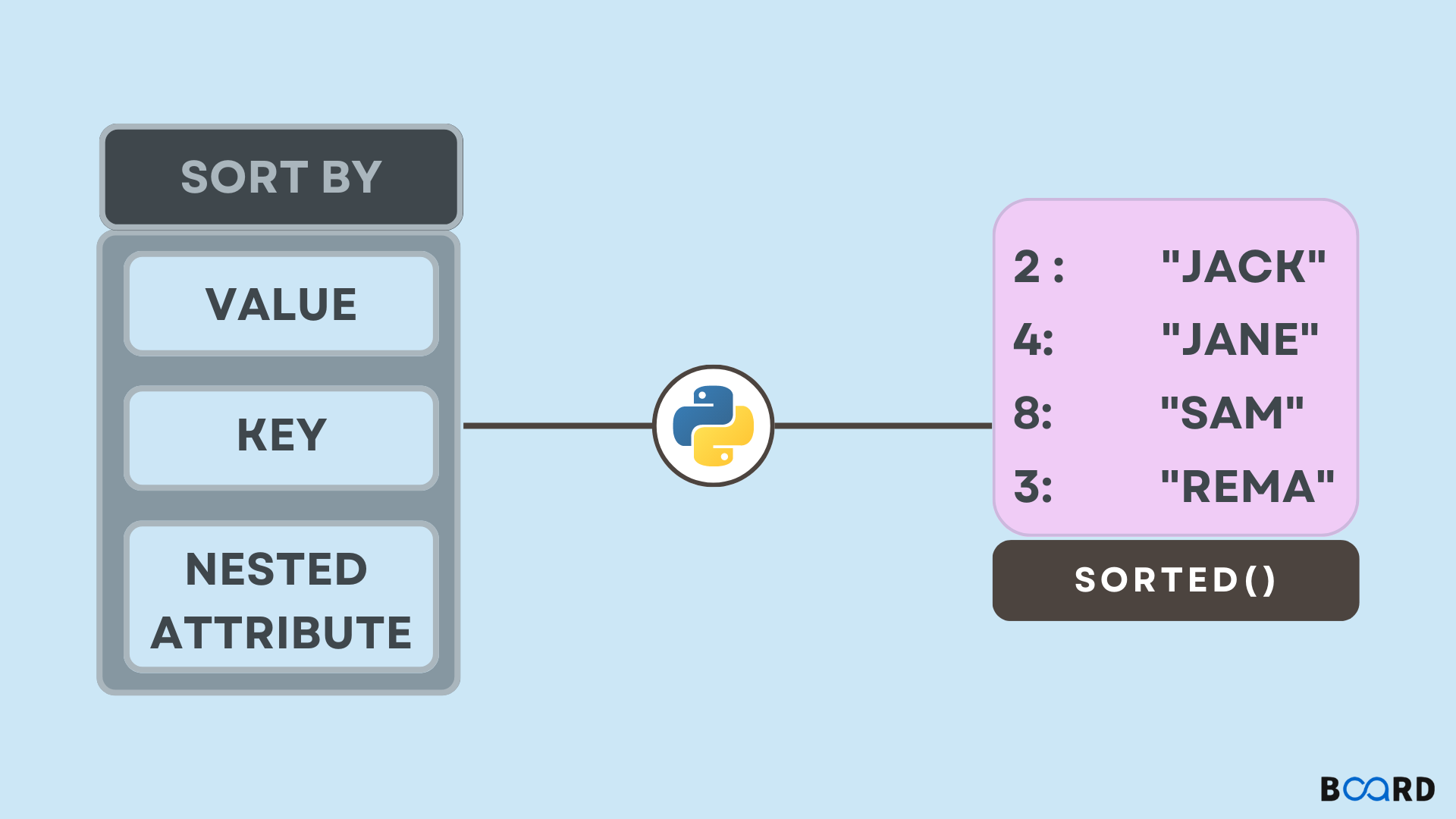# Sort Dictionary in Python## Introduction

Dictionary a Data Type in Python which stores the data in the form of key-value pairs. By default, a dictionary is unordered. All the keys in a Python Dictionary must be unique. However, the values associated with the Dictionary keys can be duplicated. But, while accessing the dictionary with duplicate values and unique keys, only one value associated with each key is printed.

See the code and its output below:

 dic= {'aa':'4', 'bb':'3','aa':'2', 'bb':'1'} for i in dic:    print(i , dic[i])

Output:

 aa 2bb 1

In this article, we will learn how to sort a Dictionary by value in Python. But, first, let’s create a Python Dictionary. Suppose dictionary keys are of character type and values associated with them are of type string. Then the program will be:

 dict= {'b':'name', 'z': "location", 'c':"contact", 'e':"roll number"}#accessing the dictionary elements in Pythonprint("Iteratively accessing dictionary ") for key in dict:    print(key, dict[key]) #another way to print all the elementsprint("Printing the dictionary ")print(dict)

Output:

 Iteratively accessing dictionary b namez locationc contacte roll numberPrinting the dictionary {'b': 'name', 'z': 'location', 'c': 'contact', 'e': 'roll number'}
write your code here: Coding Playground

## Sorting a Python Dictionary by Key

There are various ways to sort a dictionary in python. You can sort a Dictionary by value or by key in Python. To sort a dictionary by key, we can directly use the sorted method. In this method, the dictionary is first passed to the sorted()  which returns the sorted dictionary.

But, the sorted() method in Python returns a list of tuples that contains the key-value pair of the dictionary. Thus, you need to again convert the list into the tuples using the dict() method in Python.

Following is the code for sorting a dictionary by keys in python:

 dictionary= {'b':'name', 'z':"location", 'c':"contact", 'e':"roll number"} print("Printing the Sorted dictionary ")sortedDict= (sorted(dictionary.items()))#converting list of tuples into dictionary in PythonsortedDict=dict(sortedDict)print(sortedDict)

Output:

 Printing the Sorted dictionary {'b': 'name', 'c': 'contact', 'e': 'roll number', 'z': 'location'}
write your code here: Coding Playground

## Sorting a Python Dictionary by Value

You can also sort a dictionary by value in python. Suppose there is a dictionary that contains keys as numbers and values as strings. The method is the same as the previous one. The difference is that you have to pass another argument in the sorted() method which specifies the value of keys while sorting.

The key argument in the sorted() method specifies what should be considered while sorting the list. Here, we pass the lambda function as a key which specifies that the value of each key should be considered while sorting the list. The code for the above approach is below:

 #Sorting a Python dictionary by value diction= {1:'s', 2:'a', 3:'d', 4:'i'}print("Sorting Dictionary by value")sorted_dict=sorted(diction.items(), key=lambda x:x)sorted_dict=dict(sorted_dict)print(sorted_dict)

Output:

 Sorting Dictionary by value{2: 'a', 3: 'd', 4: 'i', 1: 's'}

To reverse the order of sorting by value, pass Reverse=True as argument.

 diction= {1:'s', 2:'a', 3:'d', 4:'i'}print("Sorting Dictionary by value")sorted_dict=sorted(diction.items(), key=lambda x:x, reverse=True)sorted_dict=dict(sorted_dict)print(sorted_dict)

Output:

 Sorting Dictionary by value{1: 's', 4: 'i', 3: 'd', 2: 'a'}
write your code here: Coding Playground

## Need of Sorting a Python Dictionary

Sorting any list makes retrieval of data very quick for us. The value of the data in a Python Dictionary is stored in a key-value pair. Thus, if the dictionary is sorted by key, then the value can be accessed systematically. However, it is sometimes observed that the value field in a dictionary needs to be sorted in order to make the list more systematic and organized. Therefore, if you know how to sort a dictionary by value in Python, it will make it easy for you to manipulate the data in it.